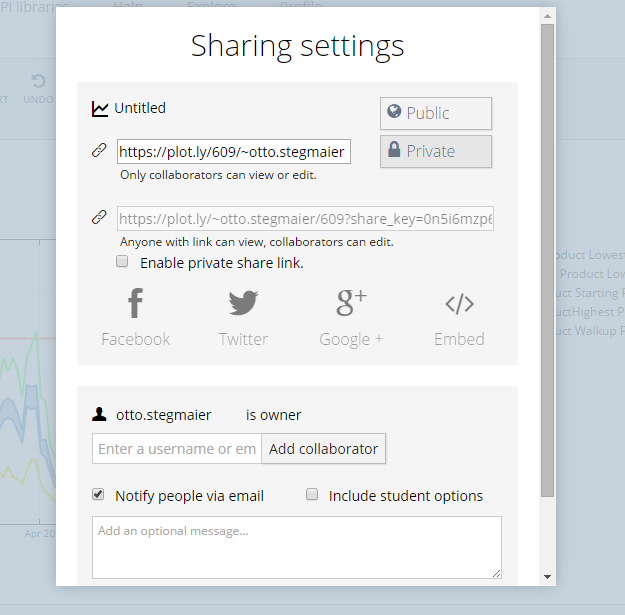Show Sidebar Hide Sidebar# Building Interactive Graphs Using Plotly and xlwings in Python/v3

Online Dashboards with Excel, Python, & Plotly

Note: this page is part of the documentation for version 3 of Plotly.py, which is not the most recent version.
See our Version 4 Migration Guide for information about how to upgrade.

# Online Dashboards with Excel, Python & Plotly¶

#### ABOUT THE AUTHOR:¶

This notebook was contributed by Plotly user Otto Stegmaier. You can follow Otto on GitHub.

#### Building Interactive Graphs at the Push of an (Excel) Button Using Plot.ly and xlwings¶

This notebook is a primer on building interacitve web-based visualizations straight from an excel workbook with

• pandas: A library with easy-to-use data structures and data analysis tools. Also, interfaces to out-of-memory databases like SQLite.
• Ipython notebook: An interface for writing and sharing python code, text, and plots.
• xlwings: A python library with tools to connect pandas to data stored in excel workbooks.
• Plot.ly: A platform for publishing beautiful, interactive graphs from Python to the web.
###### In Short... How you can go from this:¶
In :
from IPython.display import Image
Image(filename='xlwings_assets/prices.png', width = 700)

Out: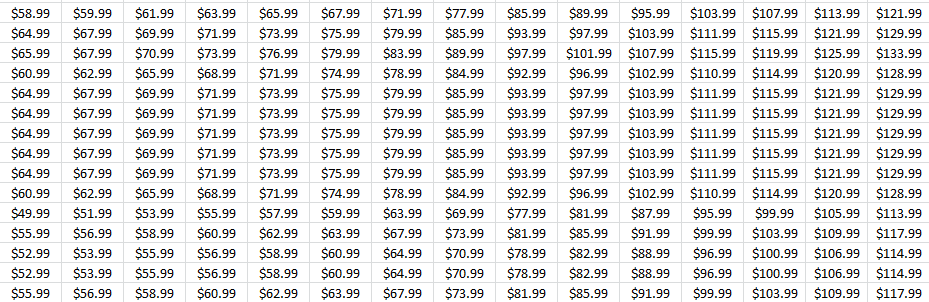###### To this:¶
In :
import plotly.tools as tls
tls.embed('https://plot.ly/~otto.stegmaier/609/previous-min-and-max-prices/')

Out:
In :
Image(filename='xlwings_assets/logo.png')

Out:###### Why we are working on this:¶

At Liftopia we are working on bringing dynamic pricing into the ski industry. We help consumers ski more by offering tickets for purchase in advance at lower prices in exchange for their commitment. We help resorts control their pricing, drive more predictable revenue and grow their businesses.

Since one of our core business channels is pricing and selling lift tickets for our resort partners, our analytics team needs to be able to communicate our pricing plans to our resort partners in a simple, but effective manner. The ski areas we work with often offer tickets on 120 days of the year at upwards of 10 different price points on each day of the season. If you do the math - that can mean trying to communicate 1,200 different prices for one product. Some resorts offer over 10 different products. Now we are at 12,000 data points. Want to see the junior and child ticket pricing too? Now that's 36,000 data points.

In an effort to communicate our pricing plans more effecitvely - we decided to use Plot.ly to help us build web based interactive visualizations we can share with our partners. To do this we connected one of our pricing tools to a python script that interacts with Plotly's API.This notebook walks through a simplified version of that process. Note - the data used in this example is intended to show how we use Plotly from Excel - if you want to talk to us about our beliefs abour pricing - get in touch! (ostegmaier@liftopia.com)

###### Covered in this notebook¶
• Connect to an excel workbook with XLWings
• Clean and prepare your data with pandas
• Plot with Plotly
• Building the VBA connection to your python code
• Sharing and Collaborating with Plotly

### Step 1: Connect to your data in Excel using xlwings¶

To show how we use plotly with XLWings and Excel - we put together some simulated data in an excel workbook. For more on XLWings Check out their documentation or this great tutorial

In :
from IPython.display import IFrame
#A few imports we will need later
from xlwings import Workbook, Sheet, Range, Chart
import pandas as pd
import numpy as np
import plotly.plotly as py
import plotly.tools as tlsM
from IPython.display import HTML
from plotly.graph_objs import *

In :
#workbook connection - When connecting to a file from a VBA macro you use Workbook.call() instead of Workbook(<filepath>)
wb = Workbook('xlwings_assets/Example Workbook.xlsm')


Using Excel as a Plotly Dashboard...

Ok - so maybe its not so high speed - but its a good fit for our users! Plotly has a ton of great GUI tools to edit the graphs once they're made, but we needed a way to make it easy on our users to get the graphs out of excel and into Plotly so they can edit the graphs there. So we built a "Dashboard" with some controls:

In :
Image(filename="xlwings_assets/dashboard.png", width="700")

Out: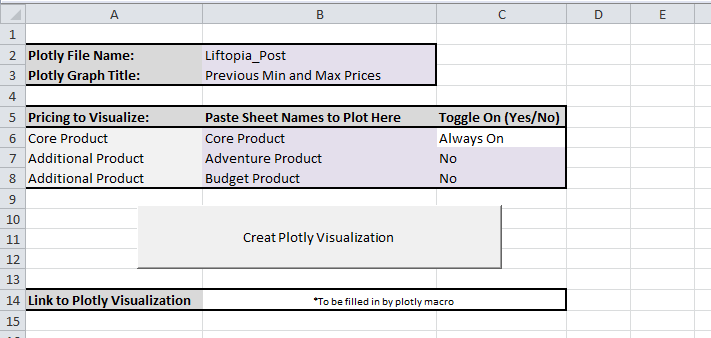Now we can use some of these user-input values to control what elements get plotted

In :
#Now we can use some of these controls to customize the
folder_name = Range('Dashboard','B2').value
graph_title = Range('Dashboard','B3').value


### Step 2: Clean and prepare your data for plotting using Pandas¶

To show how we use plotly with XLWings and Excel - we put together some simulated data in an excel workbook. For more on XLWings check out their documentation or this great tutorial

In :
#short function to create a new dataframe using xlwings
def new_df(shtnm, startcell = 'A1'):
data = Range(shtnm, startcell).table.value
temp_df = pd.DataFrame(data[1:], columns = data)
return(temp_df)

###Make some dataframes from the workbook sheets
#Core Product
shtnm1 = Range('Dashboard','B6').value
df = new_df(shtnm1)


Based on user input from the "dashboard" sheet in excel - we can choose to create a new dataframe for the 2nd product

In :
Image(filename="xlwings_assets/toggle.png", width="600")

Out: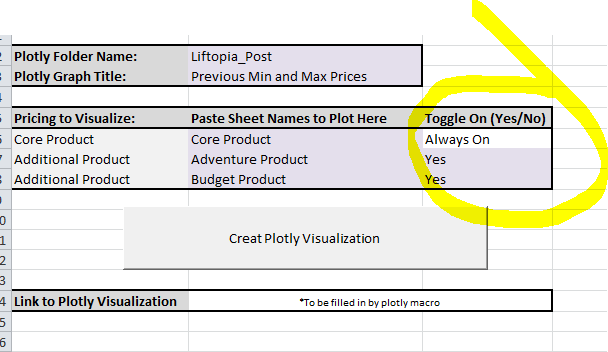In :
#2nd Product
product_2 = False
if Range('Dashboard','C7').value == "Yes":
shtnm2 = Range('Dashboard','B7').value
df2 = new_df(shtnm2)
product_2 = True

In :
#3rd Product
product_3 = False
if Range('Dashboard','C8').value == "Yes":
shtnm3 = Range('Dashboard','B8').value
df3 = new_df(shtnm3)
product_3 = True


Its easier to work with the column headers once they're cleaned up, so let's clean them up a bit

In :
#Clean up the charaters in the columns
names2 = []
def clean_names(column_list):
#Short function to make our column headers easier to reference later.
names2=[]
for name in column_list:
name = name.replace(" ","").lower()
names2.append(name)
return names2

In :
df.columns = clean_names(df.columns.values)
if product_2 == True:
df2.columns = clean_names(df2.columns.values)
if product_3 == True:
df3.columns = clean_names(df3.columns.values)


We found it useful to be using a common index across the products - at least for our purpose, so we reset the index on the date column and convert the rest of the data to float

In :
df= df.set_index('date').tz_localize('MST').astype(float)
if product_2 == True:
df2= df2.set_index('date').tz_localize('MST').astype(float)
if product_3 == True:
df3= df3.set_index('date').tz_localize('MST').astype(float)


### Step 3: Plot your data with plotly¶

In :
#set a few global variables so we can use them throughout the plots
X = df.index

try:
ymin = min(df['minpriceoffered'].min(),df2['minpriceoffered'].min(),df3['minpriceoffered'].min()) - 10
ymax = max(df['walkupprice'].max(),df2['walkupprice'].max(),df3['walkupprice'].max()) + 10

except:
#If that doesn't work, just go edit it on Plotly's web based plot editor.
ymin = df['minpriceoffered'].min() - 10
ymax = df['walkupprice'].max() + 10


For our particular use case - we were rebuilding traces of similar type, so we wrote a short function to simplify this step

In :
#function to create a "trace" (line) for each item we want to plot
def new_trace(price_column, color, name, x=X, fill = 'none', qty_column = []):
trace = Scatter(
x=X,
y=price_column,
fill=fill,
mode='lines',
name=name,
text=['Quantity: {}'.format(q) for q in qty_column],
line=Line(
color=color,
width=2,
dash='solid',
opacity=1,),
xaxis='x1',
yaxis='y1')
return trace

#Set up the 3 core traces
trace1 = new_trace(df['walkupprice'], '#FF9966','Core Product Walkup Price')
trace2 = new_trace(df['maxpriceoffered'], '#5EA5D1',shtnm1 + 'Highest Price Offered', qty_column=df['unitsmax'])
trace3 = new_trace(df['minpriceoffered'], '#5EA5D1',shtnm1+' Starting Price',  qty_column= df['unitsmin'], fill='tonexty')
trace_list = [trace1, trace2, trace3]

In :
#add additional traces if toggled on by user
if product_2 == True:   #Using the input from the Dashboard Sheet in Excel
trace4 = new_trace(df2['minpriceoffered'], '##66ff66',shtnm2+' Lowest Price Offered')
trace_list.append(trace4)

if product_3 == True:   #Using the input from the Dashboard Sheet in Excel
trace5 = new_trace(df3['minpriceoffered'], '#e6e600',shtnm3+' Lowest Price Offered')
trace_list.append(trace5)


Lastly we set some general Layout controls. If needed, these could be added as user controls pretty easily in the Excel dashboard - or you could just edit the graph from Plotly's GUI.

In :
y_axis = YAxis(
title='Price',
titlefont=Font(
size=11.0,
color='#262626'
),
range=[ymin, ymax],
domain=[0.0, 1.0],
type='linear',
showgrid=True,
zeroline=False,
showline=True,
nticks=7,
ticks='inside',
tickfont=Font(
size=10.0
),
mirror='ticks',
anchor='x1',
side='left'
)

In :
x_axis = XAxis(
title='Trip Date',
titlefont=Font(
size=11.0,
color='#262626'
),
range=[X.min(),X.max()],
domain=[0.0, 1.0],
type='date',
showgrid=True,
zeroline=False,
showline=True,
nticks=8,
ticks='inside',
tickfont=Font(
size=10.0
),
mirror='ticks',
anchor='y1',
side='bottom'
)

In :
layout = Layout(
title=graph_title,  #Using the input from the Dashboard Sheet in Excel
titlefont=Font(
size=12.0,
color='#262626'
),
showlegend=True,
hovermode='compare',
xaxis1= x_axis,
yaxis1= y_axis
)

In :
#Short function for pushing private graphs to plotly
def private_plot(*args, **kwargs):
kwargs['auto_open'] = False     #Controls whether a new tab is opened in your browser with the new plot
url = py.plot(*args, **kwargs)
return (url)


Now We are ready to plot!

In :
fig = Figure(data=trace_list, layout=layout)
url = private_plot(fig,  filename='%s/%s' %(folder_name, graph_title), sharing='public')
tls.embed(url)

Out:

### Step 4: Running your python code directly from Excel¶

• Save the python script to file. Make sure to use Workbook.caller() rather than the file path. This allows XLWings to access the current notebook that the user has open.
In :
Image(filename= 'xlwings_assets/workbookcaller.png', width="500")

Out: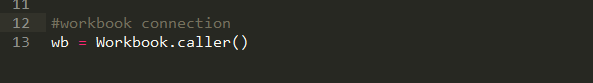• Build a Macro in python that references the script you've written:
In :
Image(filename= "xlwings_assets/macro.png", width="700")

Out: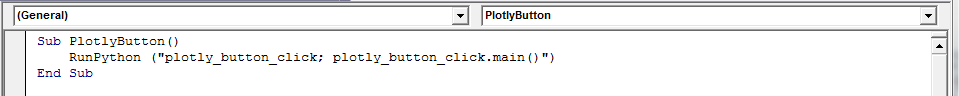• Assign the macro to a button of your choosing
In :
Image(filename= "xlwings_assets/assignmacro.png", width="500")

Out: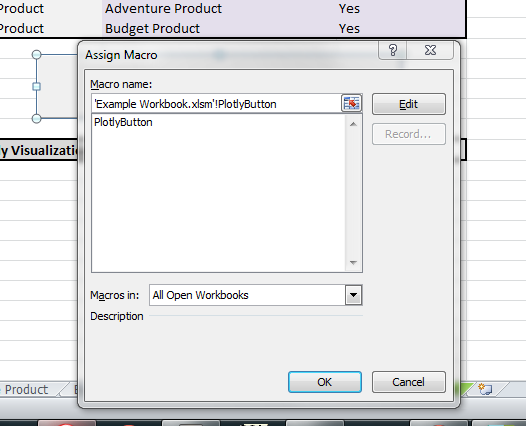• Make any final edits using Plotly's web based editor, Workspace
In :
Image(filename= "xlwings_assets/plotlyeditor.png", width="800")

Out: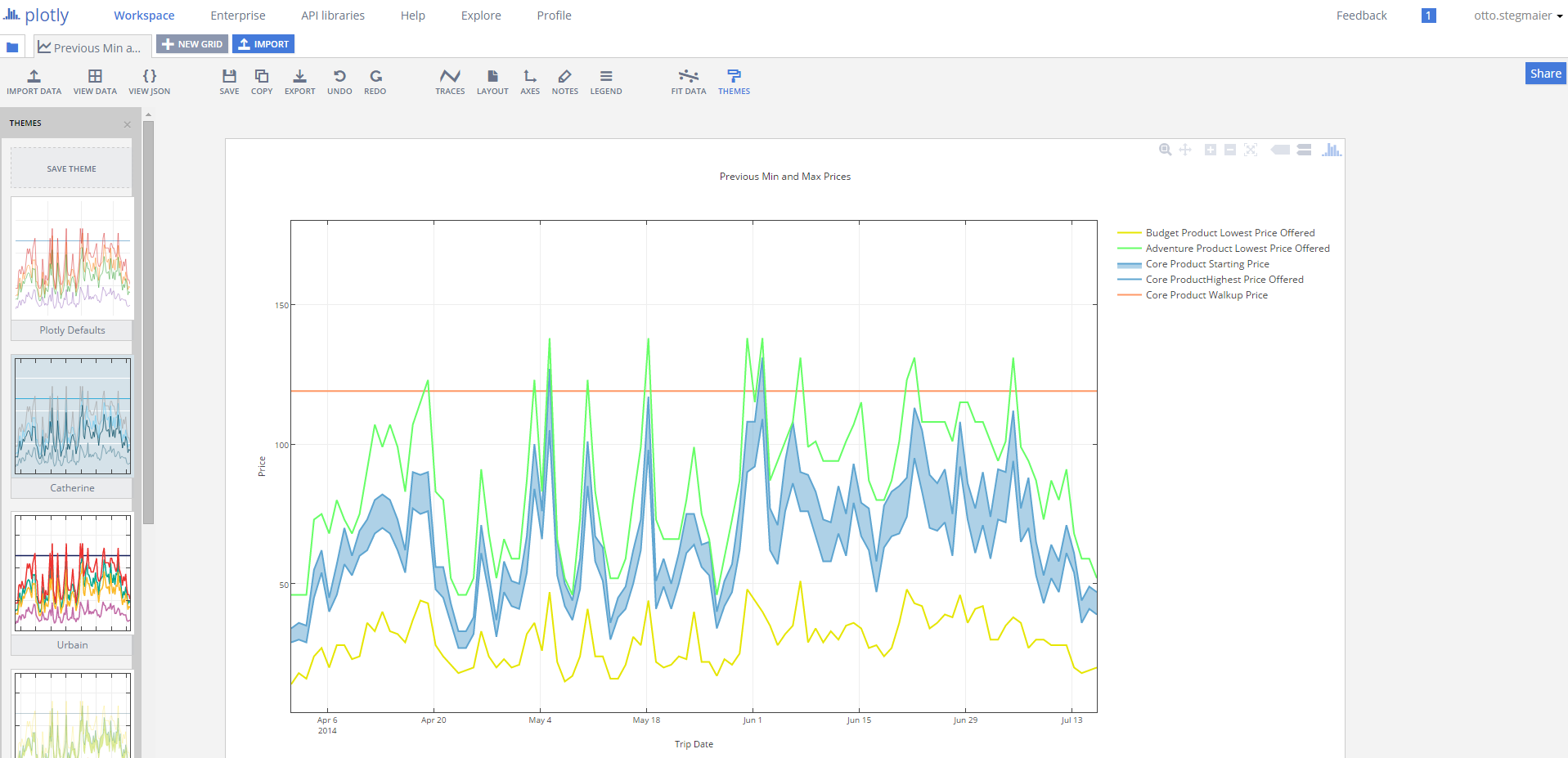### Step 5: Sharing your work and Collaborating with Others¶

One of the main reasons we wanted to use Plotly was the ability to share these interacitve visualizations via a URL. This makes it easy for our account managers to communicate the pricing plans with a simple email containging a link to the plot.

Plotly has built out some great functionality that makes sharing and collaborating really easy. When we have multiple analysts on a pricing build we can all work on a plot and once its done, its easy to share a private link with our partners. To learn more about Plotly sharing and privacy check out Plotly Getting Started and this tutorail on Plotly privacy settings

In :
Image(filename='xlwings_assets/sharing.png')

Out: